# FemtoScan Wiki

## [[en:processing:градиент]]

Trace:

Gradient is a vector showing the direction of steepest ascent of a function. Absolute value of gradient equals growth rate in this direction. Gradient filter is a difference linear filter. Difference filters including gradient filter are often referred to as edge detection filters. Unlike smoothing and contrast enhancing filters, which don’t change average intensity of image, difference filters usually provide the image with average pixel intensity close to zero. Vertical drops indicating borders of initial image regions correspond to pixel with higher intensity in processed image.

In general case directional derivative along X axis (or Y axis) for discrete function can be expressed as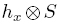(or), where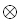refers to the resultant of discrete functions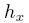and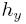and differentiable function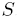(image). There are various calculation algorithms for derivative of two-dimensional discrete function. Femtoscan uses three algorithms:

• Difference algorithm. In this algorithm the X (or Y) derivative at a point is determined as the difference between values in neighboring points in line (or in column).
• Prewitt algorithm. This algorithm was created by Dr. Judith Prewitt for the most efficient edge detecting in medical images. The derivative at a point is one third from sum of differences between values in neighboring points in the same line, one line higher and one line lower (or in three columns – column with point where derivative is calculated, column to the left and column to the right).
• Sobel algorithm. This algorithm is different from Prewitt algorithm in that difference between values in neighboring points in the same line (or column) where derivative is calculated is doubled.

Gradient function can be called up from menu MathematicsLinear filters or by clicking the button on the tool bar:

When the function is called up dialog window appears with two parameters

• Algorithm determines the algorithm which will be used to calculate derivatives for gradient estimation. There are three different algorithms:
• Difference,
• Prewitt,
• and Sobel.
• Direction specifies the way of combination of two directional derivatives. It can be:
• horizontal or vertical projection of gradient (only partial directional derivative will give a contribution in this case),
• gradient projection on arbitrary vector,
• gradient magnitude, i.e. square root from the sum of the squared components.